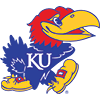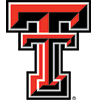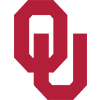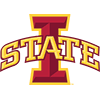DraftKings 2019 Big 12 Odds
+110
1.1 to 1Kansas
36.4% implied probability

+300
3 to 1Baylor
19.1% implied probability

+500
5 to 1Texas Tech
12.7% implied probability

+700
7 to 1West Virginia
9.5% implied probability

+1200
12 to 1Oklahoma
5.9% implied probability

+1200
12 to 1Texas
5.9% implied probability

+2000
20 to 1Iowa State
3.6% implied probability

+2500
25 to 1Oklahoma State
2.9% implied probability

+3000
30 to 1Kansas State
2.5% implied probability

+5000
50 to 1TCU
1.5% implied probability